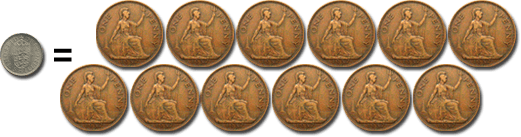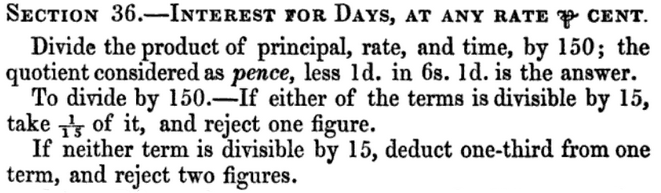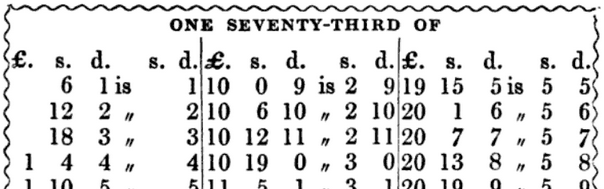# Victorian maths tricks with old money

Exploring mental arithmetic tricks in T. Martin’s 1842 guide, ‘Pounds, shillings and pence’Consider this problem from an 1869 Harvard entrance exam:That’s 50 pounds, 12 shillings and 5 pence we need to calculate 8 per cent of. Not a trivial question to work out by hand when you have to factor in how many pence there are in a shilling, as well as how many days there are in a month. Fortunately, T. Martin’s 1842 wonderful guide, Pounds, shillings and pence; or, A series of money calculations on a novel system, shows us some clever tricks which accountants such as Martin would use to work out problems like this without as much fuss as you’d think. Here, we’ll see why they work, as well.

### Twelve pence in a shilling…

Until the 1960s and 1970s, many countries which were once part of the British Empire used a currency system which was once used all over Europe. Originating from the classical Roman Empire and reintroduced to Europe in the late eighth century, the core unit was the pound (£1), which was broken up into 20 shillings (20s.):and each shilling (1s.) was broken up into 12 pence (12d.):and, if you wanted, each penny (1d.) was broken up into 4 farthings:So £1 = 20s. = 240d., and if we need to, we can have quarters of pennies as well. If you’re confused, Tom Lehrer clears it up for you. Excellent. This seems insane, of course, but in daily life, you would only be using shillings and pence. Inflation is such that £1 from 1820-1914 could buy £10 in 1973, or £100 today. Of course, accountants would be seeing mixed amounts all the time, hence Martin’s terrific guidebook.

Let’s try some of Martin’s top arithmetic tips, starting with something easy.

### A trick for multiplying shillingsMartin gives two (wordy) methods of multiplying shilling amounts here. The first method (67 × 4s.) instructs you to multiply by half the price (67 × 2 = 134), which you can then convert into pounds by taking the last digit, doubled, as shillings (4 × 2 = 8), and the rest as pounds (giving £13 8s. 0d.). This trick works because dividing by 20 (remember, 20s. = £1) is equivalent to halving and dividing by 10. Algebraically, if you have £x ys., then you have $20x + y$ shillings. Then by multiplying by half the price, you have

$\frac{20x + y}{2} = 10x + \frac{y}{2}$

in which case it’s easy to pick out x and y.

The other method is nicer mathematically: it reminds the hapless reader that multiplication is commutative! If you can’t do 67 × 4s. in your head, do 4 × 67s.! Schoolchildren were taught pence tables and so any accountant worth his salt would know 67s. = £3 7s., and this is possibly easier to multiply by 4. Just for completeness though, Martin reminds you that you can do the multiplication longhand. Remember doing multiplication with hundreds, tens and units at school? This is just like this, but with pounds, shillings and pence as your bases instead! (4 × 7 = 28 = £1 8s. Carry the 1!)

### Multiplying by 100Here’s a task which was a little more complicated fifty years ago than it is today. For common multiples, there were a whole bunch of shortcuts you could learn. Multiplying by 100 involves converting your price completely into farthings (remember, 4 farthings = 1d., so 2s. 2¾d. = 107 farthings). Taking double that number as shillings borrows from the trick we saw before, so 107s. × 2 = £10 14s. Then we add on the same number of pence, giving us £11 2s. 11d.

This works because there are 48 farthings in a shilling (in the first line), and 4 farthings in a penny (in the last line), so if you have x farthings in total, then

$100x = 48(2x) + 4x.$

You might think that performing the calculation long hand would be easier (try it… or see right!) but these tricks involve small calculations (conversions into farthings) that would be familiar to accountants of the day.

### Multiplication by division: know your aliquots!You could multiply in a more intelligent and quick way if you knew your aliquots! These are divisors of £1 or 1s. which can be written neatly in pence: Martin gives the example of 3s. 4d., which is one-sixth of a pound. He even gives a useful table of aliquot parts for shillings here, for your memorisation or to pin to your noticeboard.

Mathematically this is interesting because you are multiplying by dividing! Write your price as a sum of aliquots (here, 7½d. = 6d. + 1½d.), then

$648 \times 7\textstyle\frac{1}{2} = 648 \times (6 + 1\frac{1}{2}) = (648 \times 12) (\frac{1}{2} + \frac{1}{8}),$

which allows the method to work.

For extra fun, read Section 8, for when your price is an aliquot part of a guinea (21s.).

### Interesting interest (feat. division)Martin gives various rules for calculating interest, and this is one of my favourites, because it contains three arithmetic tricks!

Dividing by 150 and considering pounds as pence is equivalent to dividing by 150×240 = 36000. Martin has used that the rate of x% per year is equivalent to multiplying by $\frac{x}{36000}$ per day… in a 360-day year.

To help with the division by 150, the last paragraph uses the canny observation that

$\frac{xy}{150} = \frac{(\frac{2}{3}x)y}{100}.$

And finally, to fix the 360-day year problem, you have to remove 1d. for every 6s. 1d. in your answer. Why? Because

$360 = 365 – \frac{365}{73},$

and 1d. × 73 = 6s. 1d.

In case you don’t know your 6s. 1d. times table off by heart (and he recommends that you learn it), Martin provides a thorough tabulation.### The Harvard problem

Using this trick then, we shall work out the interest from the Harvard problem: £50 12s. 5d. at 8% for 5 years, 2 months and 3 days.

I’ll work out the interest for 63 days first, but I’ll let you have a go at the 5 years part.

1. Divide the product of principle, rate and time by 150:
$63 \times 8 \times {\it\unicode{xA3}} 50 \; 12\mathrm{s.} \; 5\mathrm{d.} \times 150.$
This is equivalent to multiplying by $\frac{2}{3}$ of 63 (=42) and dividing by 100, giving us
$\frac{84}{25} \times {\it\unicode{xA3}} 50 \; 12\mathrm{s.} \; 5\mathrm{d.}.$
2. The quotient considered as pence:
The above line gives us $168 \frac{42}{20}$d. ≈ 170d. = 14s. 2d.
3. Less 1d. in 6s. 1d. is the answer:
Looking at the table of seventy-thirds, the nearest entry for 14s. 2d., is 12s. 2d., so we should subtract the appropriate 2d. As our amount is two shillings away from the tabulated value, a footnote to the table allows us to subtract off a farthing, giving us a final value for the interest of 13s. 11¾d.

Section 25 shows you how to work out interest for years. I reckon the final answer should be £71 11s. 4¼d. (If you use a different definition of a month, you might get 6½d or 7d. instead)